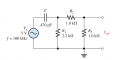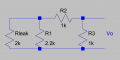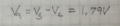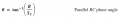# Thevenizing a leaky capacitor

#### WBahn

Joined Mar 31, 2012
25,290
So how does Floyd have you combine arbitrary impedances? Using phasor diagrams?

#### SamR

Joined Mar 19, 2019
1,835
I don't know what you mean with the left hand Z. Is it supposed to be a series combination of C and R2? If so, then that would be wrong because C is not in series with R2. To be in series, whatever current flows in one component MUST flow in the other.
OK, now that clears up a lot of my confusion since I saw the C reacting with both the parallel and series resistance.

#### SamR

Joined Mar 19, 2019
1,835
So how does Floyd have you combine arbitrary impedances? Using phasor diagrams?
OK I am burnt out for now, taking a break, more tomorrow... Thx for putting up with me!

#### The Electrician

Joined Oct 9, 2007
2,767
OK, now that clears up a lot of my confusion since I saw the C reacting with both the parallel and series resistance.
Trying to determine whether C is "reacting" with other resistances is not a technique that will be helpful when solving networks containing reactive components (capacitors and inductors).

WBahn suggested earlier "One recommendation I would make is to take some time to learn about how to work with complex numbers and then complex impedances. It really will make all of this so much easier. "

There's an old book containing many examples, and working examples is what will increase your skill level. I worked most of the examples in this book decades ago, using a slide rule. You can buy a used copy for under \$10:

•SamR

#### SamR

Joined Mar 19, 2019
1,835
I spent a couple more hours on it and still not done. At this point, Floyd is introducing RC circuits and going into the phase relationships, introducing Impedance and it's triangle, Lag and Lead RC, Conductance, Susceptance and Admittance and touching on filtering and bandwidth. Barely touching on the combination of Parallel and Series Impedance by using series equivilants. No complex equations although bringing in the theta and iota angles and the introduction of radians (which is not new to me). I like this book because Floyd brings into play some application and problem solving along with the basic theory. He does (and this is a good example) occasionally throw a problem at you that is a poser and requires you to pull it all together in order to solve. Not just a recitation/regurgitation of the current subjects examples given and worked through. It is challenging for me and I enjoy the mental gymnastics but can lose focus easily when tired which at my age is often.

#### SamR

Joined Mar 19, 2019
1,835

#### SamR

Joined Mar 19, 2019
1,835
So it wants the Thevenin equivalent circuit of:Which by replacing the C with Rleak becomes:Now since they have flipped the capacitor in the circuit from that of the example I am confused... Do I remove Rleak and Thevenize as from Vo Rth = R3||R1+R2 or from Rleak Rth = R1||R2+R3? The example is given as a Rth||Rleak and here it seems to be in series?

#### MrAl

Joined Jun 17, 2014
7,179
There isn't one Jony, hence this thread. I tried to find one using LTS but am not getting the same answer.
Hi,

If you want to put things in parallel that have resistance and reactance you are better off using complex impedances because then the math follows the same basic rules as for resistors. This leads to a lot of tricks, but even without that it gets more straightforward.

Previously you wanted to place a resistor in parallel with a capacitor.
In terms of the Laplace variable 's' this would be:
zC=1/(s*C)
zR=R

and now for the parallel combo you know that two resistors result in:
RT=R1*R2/(R1+R2)

well for the R and C it becomes:
ZT=zR*zC/(zR+zC)

and of course the zR's are simple R:
ZT=R*zC/(R+zC)

and the math is still fairly simple:
ZT=R*1/(s*C)/(R+1/(s*C))

multiply top and bottom by s*C and get:
ZT=R/(s*R*C+1)

and now you can replace 's' with 'jw' (jw=j*w) and get:
ZT=R/(jw*R*C+1)

and now convert this to a form with all reals in the denominator by multiplying top and bottom by the complex conjugate of the denominator which is:
1-jw*R*C

ZT=R*(1-jw*R*C)/((1+jw*R*C)*(1-jw*R*C))

and the result is:
R-j*w*C*R^2/(w^2*C^2*R^2+1)

where you can see the denominator is now all reals, and separating the two parts:
R/(w^2*C^2*R^2+1) is the real part of the result, and
w*C*R^2/(w^2*C^2*R^2+1) is the imaginary part of the result.

When you are working with all known quantities this results in:
a+b*j

which is just one real number and one imaginary number added together like 1.432+2.41844*j.
That result can then be used in the next calculation the way any complex number is used.

The amplitude is then:
sqrt(a^2+b^2)

and the phase angle is:
atan2(b,a)

The beauty of this is no matter how complicated the circuit is you can use complex numbers to solve it.
Of course a math software goes well with this, one that can handle complex quantities.
I always recommend this because of its wide applicability and using reactances gets old and hard to handle really really fast.

#### SamR

Joined Mar 19, 2019
1,835
Al I appreciate that but at this point, I am trying to understand what is being given in the textbook. I thought I was pretty good at Thevenizing but this pi resistor arrangement has me stumped it seems. I can't find a text describing how to handle it.

#### MrAl

Joined Jun 17, 2014
7,179
Al I appreciate that but at this point, I am trying to understand what is being given in the textbook. I thought I was pretty good at Thevenizing but this pi resistor arrangement has me stumped it seems. I can't find a text describing how to handle it.
Did you investigate something like Nodal Analysis or similar yet?
You need a general way to handle networks or you will be stumped with each new network.
We all face new networks from time to time, so having a general method on hand we can quickly derive the quantities we need to know in order to understand the circuit, and pretty soon we figure out what we have to look for. It does get easier.

#### SamR

Joined Mar 19, 2019
1,835
Did you investigate something like Nodal Analysis or similar yet?
Not as yet. Still working on DC & AC fundamentals. Still on the to-do list are Malvino's "Resistive and Reactive Circuits" and Floyd's "Electronic Devices".

#### WBahn

Joined Mar 31, 2012
25,290
Al I appreciate that but at this point, I am trying to understand what is being given in the textbook. I thought I was pretty good at Thevenizing but this pi resistor arrangement has me stumped it seems. I can't find a text describing how to handle it.
The approach they are driving you towards is to look at the circuit from the capacitor's point of view. Doing that let's you find a Thevenin equivalent for the rest of the circuit that is purely resistive. With that, you can then find the voltage across the capacitor. See if that helps.

•SamR

#### The Electrician

Joined Oct 9, 2007
2,767
The approach they are driving you towards is to look at the circuit from the capacitor's point of view. Doing that let's you find a Thevenin equivalent for the rest of the circuit that is purely resistive. With that, you can then find the voltage across the capacitor. See if that helps.
Your comment seems directed toward the circuit shown in post #47, but SamR is actually working on the circuit in post #48.

#### WBahn

Joined Mar 31, 2012
25,290
Your comment seems directed toward the circuit shown in post #47, but SamR is actually working on the circuit in post #48.

My thinking is that if you were given the voltage (magnitude and phase) of the voltage across the capacitor, then you should be able to determine the voltage across the resistive side of the network. Once you know that, it is trivial to find the output voltage. So how might we find the voltage across the capacitor? Treat IT as a load and thevenize everything else.

#### The Electrician

Joined Oct 9, 2007
2,767
So it wants the Thevenin equivalent circuit of:
The problem statement you posted in post #41 doesn't require you to use Thevenin's theorem, so why are you trying to use it? As just a voltage divider problem it's easier.

#### WBahn

Joined Mar 31, 2012
25,290
I tried the Thevenin method and here is what I am getting, close but not exact...
View attachment 202401
Unless someone sees some egregious errors, I'm calling this close enuff...
You are fine up to this point:You cannot just subtract these two voltages arithmetically because they are NOT in phase.

This IS a very egregious error and the fact that the result is anywhere close to the actual answer is pure coincidence. I could (probably) easily change circuit so that you answer using this approach would be off by an order of magnitude.

You cannot ignore the phase relationships in these circuits!

Consider the Thevenin circuit that has a series resistor of 687.5 Ω and a capacitive reactance of -3386 Ω.

What is the angle of the resulting impedance?

θ = Atan(-3386 Ω / 687.5 Ω) = -78.52 °

The phase of the current flowing through the Thevenin circuit (and hence the capacitor) is thus +78.52°

The phase of the voltage across the capacitor is then 78.52° - 90° = -11.48°.

So the in-phase component of the voltage is

Vinphase = 3.215 V cos(θ) = 3.151 V

and the out-of-phase component is

Voutphase = 3.215 V sin(θ) = -0.6399 V

When you subtract this voltage from the 5 V (all of which is in-phase since it is our reference), you have to subtract the in-phase components separately from the out-of-phase components.

So the in-phase component of the voltage at Va:

Va_inphase = 5 V - 3.151 V = 1.849 V

while the out-of-phase component of Va is

Va_outphase = 0 V + 0.6399 V = 0.6399 V

The amplitude of the voltage at Va is the Pythagorean sum of these two:

|Va| = 1.9566 V

And Vo is half of this, or 978 mV

There are all kinds of opportunities to mess up these relationships. Working with complex impedances eliminates the vast majority of them because it tracks them for you.

#### SamR

Joined Mar 19, 2019
1,835
There are all kinds of opportunities to mess up these relationships. Working with complex impedances eliminates the vast majority of them because it tracks them for you.
I remember that very well from going through Grob's.
I tried a different method that also did not work. Using the series Requivalent for C and Rleak.OK still working over the notes from your last post, more later.

Thanks, Sam

#### SamR

Joined Mar 19, 2019
1,835
Consider the Thevenin circuit that has a series resistor of 687.5 Ω and a capacitive reactance of -3386 Ω.
I calculated Rth as Rleak||R1||(R2+R3) = 687.5Ω

I calculated Xc as 1/(2πfC) = 3388Ω So I am at a loss as to where -3386Ω comes from?So I have Θ = arctan(687.5/3388) = 11.47°

OK from your notes I do see what you are doing, but it is outside of the material being presented in this introductory chapter to RC. Which is why using the information given in this chapter I am unable to match the output from LTS. The closest I can come seems to be the conversion of the R||C to it's Requivalent for a series voltage divider. And that is not close to being correct.

Time to pigeonhole this problem and move on... I may revive it after Floyd covers complex equations.